Test: Strength of Materials - 2

# Test: Strength of Materials - 2 - Mechanical Engineering

Test Description

## 25 Questions MCQ Test Mechanical Engineering SSC JE (Technical) - Test: Strength of Materials - 2

Test: Strength of Materials - 2 for Mechanical Engineering 2023 is part of Mechanical Engineering SSC JE (Technical) preparation. The Test: Strength of Materials - 2 questions and answers have been prepared according to the Mechanical Engineering exam syllabus.The Test: Strength of Materials - 2 MCQs are made for Mechanical Engineering 2023 Exam. Find important definitions, questions, notes, meanings, examples, exercises, MCQs and online tests for Test: Strength of Materials - 2 below.
Solutions of Test: Strength of Materials - 2 questions in English are available as part of our Mechanical Engineering SSC JE (Technical) for Mechanical Engineering & Test: Strength of Materials - 2 solutions in Hindi for Mechanical Engineering SSC JE (Technical) course. Download more important topics, notes, lectures and mock test series for Mechanical Engineering Exam by signing up for free. Attempt Test: Strength of Materials - 2 | 25 questions in 50 minutes | Mock test for Mechanical Engineering preparation | Free important questions MCQ to study Mechanical Engineering SSC JE (Technical) for Mechanical Engineering Exam | Download free PDF with solutions
 1 Crore+ students have signed up on EduRev. Have you?
Test: Strength of Materials - 2 - Question 1

### If a column is changed from both ends fixed toboth ends hinged its load carrying capacity .

Test: Strength of Materials - 2 - Question 2

### Shown below are two cross sections of two shafts 1 and 2. The ratio of maximum torque carring capacity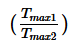. If they are made of same material is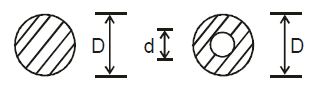d/D = 1/2

Test: Strength of Materials - 2 - Question 3

### The maximum deflection of a simply supported beam due to its self weight (W) is.

Test: Strength of Materials - 2 - Question 4

It flexural strength decreases the maximum slopeof any beam

Test: Strength of Materials - 2 - Question 5

If temperature of a freely hanging bar is increasedit will develope : -

Test: Strength of Materials - 2 - Question 6

Find the increase in temperature (DT) so that the gap gets filled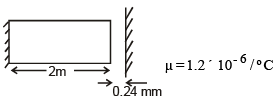Test: Strength of Materials - 2 - Question 7

The ratio of hoop stress for a thin cylinder andthin spherical pressure vessel is

Test: Strength of Materials - 2 - Question 8

A spherical pressure vessel is to be designed tohold 40 MPa pressure and Diameter should be200 mm. If max stress can be 200 MPa find thethickness required.

Test: Strength of Materials - 2 - Question 9

Shown below is a B.M.D.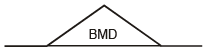The SFD will be

Test: Strength of Materials - 2 - Question 10

Shown is a B.M.D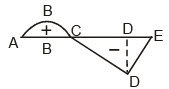The shear force is zero at

Test: Strength of Materials - 2 - Question 11

Shown is a B.M.DIn the above diagram point of contraflexure is

Test: Strength of Materials - 2 - Question 12

In the above shown BMD, the beam is mostprobably

Test: Strength of Materials - 2 - Question 13

Strain Energy for the shown beam is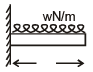Test: Strength of Materials - 2 - Question 14

The B.M.D. for a cantilever beam due to its selfweight is

Test: Strength of Materials - 2 - Question 15

For a structural member the principle stressesare equal but opposite in nature . The center ofmohr's circle will

Test: Strength of Materials - 2 - Question 16

The maximum shear stress in a mohr's circle isequal to

Test: Strength of Materials - 2 - Question 17

A spring of length 'L' is cut into 5 equal parts if initial spring stiffness was R the final stiffness after
parallel combination of all 5 parts will be

Test: Strength of Materials - 2 - Question 18

It E is the young's Modulus, the minimum value of bulk modulus is

Test: Strength of Materials - 2 - Question 19

It the dimentions of a prismatic bar hanging fromroof are doubled, the elongation due to its selfweight will become

Test: Strength of Materials - 2 - Question 20

A long shaft of diameter d is subjected to twisting moment of T. The maximum normal stress acting
at the cross- section is equal to :-

Test: Strength of Materials - 2 - Question 21

For shafts in parallel which of the following willbe same for all shafts.

Test: Strength of Materials - 2 - Question 22

How many minimum values among E, G, K, Mwould you require for a isotropic andhomogenous material to determine all state ofstress.

Test: Strength of Materials - 2 - Question 23

The maximum shear stress in a shaft of Diameter100 mm is 100MPa. The shear stress at adistance of 30 mm from center is .

Test: Strength of Materials - 2 - Question 24

The depth of a beam of uniform strength vary : -

Test: Strength of Materials - 2 - Question 25

Which of the following is must for a strut.

## Mechanical Engineering SSC JE (Technical)

6 videos|97 docs|57 tests
Information about Test: Strength of Materials - 2 Page
In this test you can find the Exam questions for Test: Strength of Materials - 2 solved & explained in the simplest way possible. Besides giving Questions and answers for Test: Strength of Materials - 2, EduRev gives you an ample number of Online tests for practice

## Mechanical Engineering SSC JE (Technical)

6 videos|97 docs|57 tests(Scan QR code)